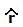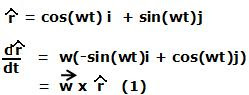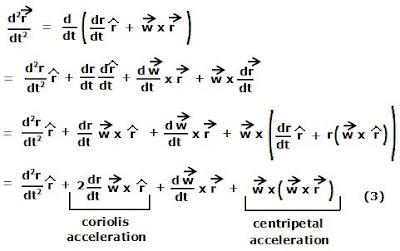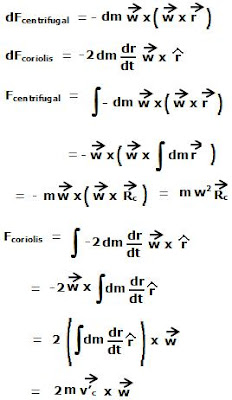Thursday, February 12, 2009

Irodov Problem 1.268Letbe a certain radius vector of a point rotating with an angular velocity vector. Let the unit vector in the direction ofbe. Let us choose and x,y,z Cartesian coordinate system such that the z-axis aligns with the angular velocity vector and the point rotates in the x,y plane. Then we have,In Eqn (1) w is the magnitude of the angular velocity vector. Let r be the magnitude of, then we have,Differentiating once again,When seen from the rotating frame both these acceleration appear as pseudo forces with a negative sign. Now consider a infinitesimally small piece of the rotating body of mass dm. The centrifugal force and the Coriolis force experienced by this mass are respectively given by,